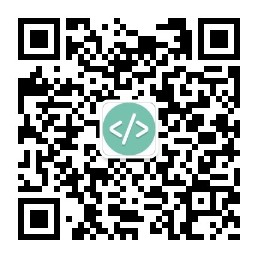# LeetCode每日一题: 亲密字符串（No.859）

```给定两个由小写字母构成的字符串 A 和 B 。

### 示例：

```输入： A = "ab", B = "ba"

### 思考：

```这道题首先判断两个字符串长度是否相等，长度不相等不可能是亲密字符串。

### 实现：

```class Solution {
public boolean buddyStrings(String A, String B) {
//比较长度
if (A.length() != B.length()) {
return false;
}
//字符串是否相等
if (A.equals(B)) {
//相等判断是否有重复字符
for (int count = 0; count < A.length(); count++) {
if (A.indexOf(A.charAt(count), count + 1) != -1) {
//有重复字符返回true
return true;
}
}
//没有返回false
return false;
} else {//字符串不相等
//记录不相等字符出现次数
int time = 0;
//记录不相等字符位置
List<Integer> pos = new ArrayList<>();
for (int count = 0; count < A.length(); count++) {
if (A.charAt(count) != B.charAt(count)) {
time++;
}
}
//次数不等于2 不是亲密字符串
if (time !=2) {
return false;
} else {
//等于2交换字符后再次比较
return swap(A, pos.get(0), pos.get(1)).equals(B);
}
}
}
private String swap(String str, Integer pos1, Integer pos2) {
char[] charArray = str.toCharArray();
char temp = charArray[pos1];
charArray[pos1] = charArray[pos2];
charArray[pos2] = temp;
return new String(charArray);
}
}复制代码```### 相关软件推荐:

#### 今日简史

[以] 尤瓦尔·赫拉利 / 林俊宏 / 中信出版集团 / 2018-8 / 68